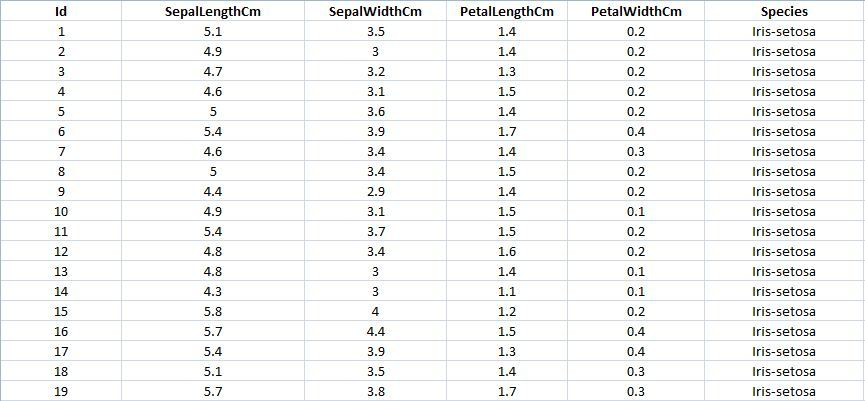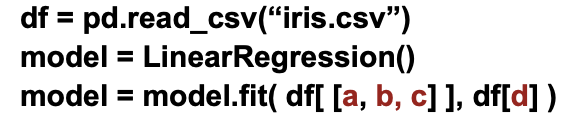# Machine Learning Models in Python with sk-learn

The easiest to use library to start working on machine learning in Python is using a library called scikit-learn (or commonly just "sk-learn"). Part of the simplicity of this library is that the same functions and steps are used for all different types of machine models learning! Specifically, we will always follow four steps:

1. Import the library for the specific machine learning model we want to use,

2. Create an instance of the model,

3. Train the model using model.fit(...)

4. Use the model by using model.predict(...), using the model we trained in the previous step to predict the outcome

## Example: Linear Regression

Linear Regression is one of the easiest to understand machine learning models, where Python will use the training data to find a "line of best fit" to predict the outcome. The sk-learn linear regression model is sklearn.linear_model.LinearRegression, which means our import line would be:

# Step 1 - Import the library:
from sklearn.linear_model import LinearRegression

The second step will always be to create a new instance of the model, which we'll call model:

# Step 2 - Create an instance of the model:
model = LinearRegression()

The third step is requires us to train our model. In this example, we will use the diamonds dataset to predict the price based on the size (carat weight) of the diamond. To do this:

• We use a pandas DataFrame to load the data,

• We specify the independent variables as list of values -- here we only have the carat weight, so we have a list of one element: ["carat"]

• We specify the dependent variable directly (as LinearRegression allows only a single dependent variable): "price"

# Step 3 - Train the model:
import pandas as pd
model = model.fit( df[ ["carat"] ], df["price"] )

Finally, in the final step, we can predict the price of a diamond by providing a list of independent variables. We can do this by creating a simple DataFrame and using the model to predict the price:

# Step 4 - Predict the outcome:

# Create a new DataFrame from scratch to predict the price of a 1, 2, and 3 ct.
# diamond.  We'll call this df2:
data = []
data.append( {"carat": 1} )
data.append( {"carat": 2} )
data.append( {"carat": 3} )
df2 = pd.DataFrame(data)

# Add a new column to df2 with the predicted prices:
df2["price_predict"] = model.predict( df2 )

Putting this all together:

# Step 1 - Import the library:
from sklearn.linear_model import LinearRegression
# Step 2 - Create an instance of the model:
model = LinearRegression()
# Step 3 - Train the model:
import pandas as pd
model = model.fit( df[ ["carat"] ], df["price"] )
# Step 4 - Predict the outcome:
# Create a new DataFrame from scratch to predict the price of a 1, 2, and 3 ct.
# diamond.  We'll call this df2:
data = []
data.append( {"carat": 1} )
data.append( {"carat": 2} )
data.append( {"carat": 3} )
df2 = pd.DataFrame(data)
# Add a new column to df2 with the predicted prices:
df2["price_predict"] = model.predict( df2 )

# Display df2 to see our results:
df2
caratprice_predict
015500.065038
1213256.490656
2321012.916274

Complete Linear Regression source code.

# Example Walk-Throughs with Worksheets

### Video 1: Simple Linear Regression in Python

Follow along with the workseet to work through the problem:

### Video 2: Multiple Linear Regression in Python

Follow along with the workseet to work through the problem:

# Practice Questions

Q1: Which of the following is correct?
Q2: The DataFrame in the image is the Iris dataset. We want to predict PetalLengthCm( petal length in centimeters) from three other independent variables SepalLengthCm, SepalWidthCm and PetalWidthCm. Which variable should be placed in the place of d in the following snippet of code?